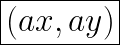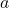## what coordinate expression represents the coordinates of any point after dilation by any scale factor, a?

Question

what coordinate expression represents the coordinates of any point after dilation by any scale factor, a?

in progress 0
2 months 2021-07-22T13:29:17+00:00 2 Answers 10 views 0

(x,y) → (ax,ay) .

Step-by-step explanation:

A dilation is a transformation that changes the size of the image. It either shrink or enlarge a shape by using a scale factor about a center of dilation.

If we transform point (x,y) by using dilation with scale factor k (assume center of dilation at (0,0) then the coordinate of the image will be (kx,ky).

So, the coordinate expression represents the coordinates of any point after dilation by a scale factor of a:

(x,y) → (ax,ay) .In order to dilate the coordinates of a point, we want to make ittimes bigger. In order to do this, we can multiply the x and y values by our scale factor, a. This is called mapping. What it does is it basically multiplied our points by a specific number so that we end up with a figure that is(the scale factor) times bigger than the original.